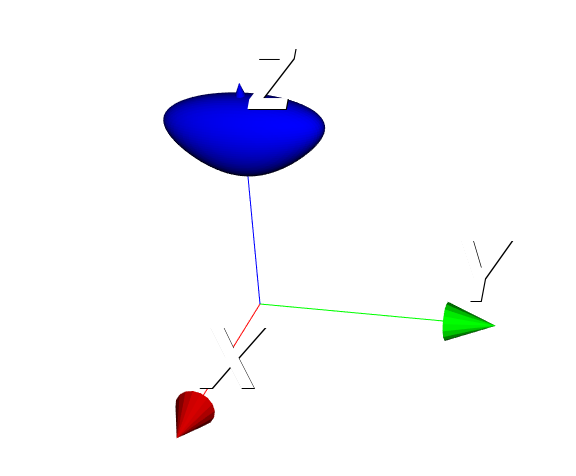# Looking for a Python VTK equivalent to Matlab's Isosurface function

I have the data as a (n_x*n_y*n_z) array in Matlab, which I can easily transfer over to numpy using scipy.io.

My code so far looks like this:

``````    frommat = loadmat("thefile.mat")

vtk_data_array = numpy_support.numpy_to_vtk(num_array=export.ravel(), deep=True, array_type=vtk.VTK_FLOAT)

img_vtk = vtk.vtkImageData()
img_vtk.SetDimensions(export.shape)
img_vtk.GetPointData().SetScalars(vtk_data_array)

implicit_volume = vtk.vtkImplicitVolume()
implicit_volume.SetVolume(img_vtk)

thresholder = vtk.vtkImageThreshold()
thresholder.SetInputData(img_vtk)
thresholder.ThresholdByLower(isosurf_value)
thresholder.ReplaceInOn()
thresholder.SetInValue(0) #<- not sure what these do
thresholder.ReplaceOutOn()
thresholder.SetOutValue(1) #<- not sure what these do
thresholder.Update()

dmc = vtk.vtkDiscreteMarchingCubes()
dmc.SetInputConnection(thresholder.GetOutputPort())
dmc.GenerateValues(1, 1, 1) #<- not sure what these do
dmc.Update()

mapper = vtk.vtkPolyDataMapper()
mapper.SetInputConnection(dmc.GetOutputPort())

actor = vtk.vtkActor()
actor.SetMapper(mapper)

``````

But in matlab, I just run

`````` surface = isosurface(the_3d_data, isosurf_value);
``````

to get the same thing.

I’m kinda new to vtk so I’m not sure how to properly use vtkDiscreteMarchingCubes, but I know that’s the key function here.

References:

There is no need for thresholding. You can use vtkContourFilter directly on the input volume.

If you want to see less of VTK pipelines and instead use VTK via a more Pythonic wrapper then you can use PyVista.

I removed the thresholder part to this:

``````    img_vtk = vtk.vtkImageData()
img_vtk.SetDimensions(export.shape)
img_vtk.GetPointData().SetScalars(vtk_data_array)

implicit_volume = vtk.vtkImplicitVolume()
implicit_volume.SetVolume(img_vtk)

contours = vtk.vtkContourFilter()
contours.SetInputData(img_vtk)
contours.GenerateValues(8, 0.0, 1.2)

dmc = vtk.vtkDiscreteMarchingCubes()
dmc.SetInputData(contours)
dmc.GenerateValues(1, 1, 1)
dmc.Update()

mapper = vtk.vtkPolyDataMapper()
mapper.SetInputConnection(dmc.GetOutputPort())

actor = vtk.vtkActor()
actor.SetMapper(mapper)
``````

But I’m getting an error with vtk.vtkDiscreteMarchingCubes(), it says

``````dmc.SetInputData(contours)
TypeError: SetInputData argument 1: method requires a vtkDataObject, a vtkContourFilter was provided.
``````

I also tried changing SetInputData to SetInputConnection but I don’t know if that’s the right approach… I think I’m missing something very fundamental.

This looks better, but you can also remove vtkImplicitVolume and vtkDiscreteMarchingCubes filters. You just need image data -> contour filter -> poly mapper. See this example: https://kitware.github.io/vtk-examples/site/Python/Visualization/FrogReconstruction/

The code runs, but I’m not getting my expected result.

This is what I get:

and my expected result looks like this: (I’m drawing it in VTK through an exported STL)My code looks like this now

``````    frommat = loadmat("matlab_file.mat")
export = frommat["export"]
isosurf_value = frommat["isosurf_value"]

vtk_data_array = numpy_support.numpy_to_vtk(num_array=export.ravel(), deep=True, array_type=vtk.VTK_FLOAT)

img_vtk = vtk.vtkStructuredPoints()
img_vtk.SetDimensions(export.shape)
img_vtk.GetPointData().SetScalars(vtk_data_array)

contours = vtk.vtkContourFilter()
contours.SetInputData(img_vtk)
contours.SetValue(0, isosurf_value)

mapper = vtk.vtkPolyDataMapper()
mapper.SetInputConnection(contours.GetOutputPort())

actor = vtk.vtkActor()
actor.SetMapper(mapper)

_axes_actor = vtk.vtkAxesActor()
``````

The isosurf value I’m using in the code above is the same one I used in the matlab code.

Maybe I’m missing something with the numpy export…?

Numpy array shape is not the same as image dimensions: axis order is reversed in numpy arrays compared to C++ toolkits, such as VTK or ITK.

I slapped a [::-1] and made the shape

``````img_vtk.SetDimensions(export.shape[::-1])
``````

and now it works! Thank you very much.

1 Like